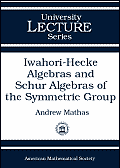## Iwahori-Hecke algebras and Schur algebras of the symmetric group

### Andrew Mathas

University lecture series, 15, American Mathematical Society (Providence, R.I.), 1999.
MR 2001g:20006

#### Key words

Iwahori-Hecke algebras, q-Schur algebras, cellular algebras.

#### Abstract

This volume presents a fully self-contained introduction to the modular representation theory of the Iwahori-Hecke algebras of the symmetric groups and of the q-Schur algebras. The study of these algebras was pioneered by Dipper and James in a series of landmark papers. The primary goal of the book is to classify the blocks and the simple modules of both algebras. The final chapter contains a survey of recent advances and open problems.

The main results are proved by showing that the Iwahori-Hecke algebras and q-Schur algebras are cellular algebras (in the sense of Graham and Lehrer). This is proved by exhibiting natural bases of both algebras which are indexed by pairs of standard and semistandard tableaux respectively. Using the machinery of cellular algebras, which is developed in Chapter 2, this results in a clean and elegant classification of the irreducible representations of both algebras. The block theory is approached by first proving an analogue of the Jantzen sum formula for the q-Schur algebras.

This book is the first of its kind covering the topic. It offers a substantially simplified treatment of the original proofs. The book is a solid reference source for experts. It will also serve as a good introduction to students and beginning researchers since each chapter contains exercises and there is an appendix containing a quick development of the representation theory of algebras. A second appendix gives tables of decomposition numbers.

• Introduction (dvi or pdf)
• Chapter 1: The Iwahori-Hecke algebra of the symmetric group
• The Symmetric group
• The Iwahori-Hecke algebra
• The 0-Hecke algebra
• Chapter 2: Cellular algebras
• Cellular bases
• Simple modules in a cellular algebra
• Chapter 3: The modular representation theory of H
• The combinatorics of tableaux
• The Murphy basis
• Specht modules and Jucys-Murphy elements
• Irreducible H-modules
• Chapter 4: The q-Schur algebra
• Semistandard tableaux
• A Specht filtration of Mmu
• The semistandard basis theorem
• Chapter 5: The Jantzen sum formula and the blocks of H
• Gram determinants of Weyl modules
• The Jantzen sum formula
• The blocks of S(n) and H
• Irreducible Weyl modules and Specht modules
• Chapter 6: Branching rules, canonical bases and decomposition matrices
• The LLT algorithm
• Decomposition maps and adjustment matrices
• The Kleshchev-Brundan modular branching rules
• Rules for computing decomposition matrices
• The q-Schur algebras and GLn(q)
• The Ariki-Koike algebras and cyclotomic q-Schur algebras
• Appendix A: Finite dimensional algebras over a field
• Filtrations and composition series
• Idempotents and indecomposable modules
• The blocks of A
• Semisimple symmetric algebras
• Appendix B: Decomposition matrices
• Decomposition matrices when e=2
• Decomposition matrices when e=3
• Decomposition matrices when e=4
• Appendix C: Elementary divisors of integral Specht modules
• Index of notation
• References
• Index

ISBN 0-8218-1926-7. 211 pages.

#### AMS Subject Classification (1991)

Primary: 20C30, 16G99
Secondary: 05E10, 20G05, 20C20

Andrew Mathas
August 1999
.